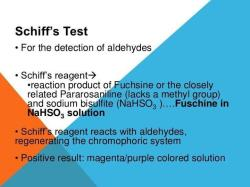Courses

Test: Periodic Scheduling

15 Questions MCQ Test Embedded Systems (Web) | Test: Periodic Scheduling

Description
Attempt Test: Periodic Scheduling | 15 questions in 25 minutes | Mock test for Computer Science Engineering (CSE) preparation | Free important questions MCQ to study Embedded Systems (Web) for Computer Science Engineering (CSE) Exam | Download free PDF with solutions
QUESTION: 1

The execution of the task is known as

Solution:

Explanation: The execution of the task is known as the job. The time for both the execution of the task and the corresponding job is same.

QUESTION: 2

Which scheduling algorithm is can be used for an independent periodic process?

Solution:

Explanation: The RMS os rate monotonic scheduling is periodic scheduling algorithm but EDD, LL, and LST are aperiodic scheduling algorithm.

QUESTION: 3

What is the relationship between the priority of task and their period in RMS?

Solution:

Explanation: The priority of the task decreases monotonically with respect to their period in the rate monotonic scheduling, that is, the task with the long period will get a low priority but task with the short period will get a high priority.

QUESTION: 4

Which of the following uses a preemptive periodic scheduling algorithm?

Solution:

Explanation: The rate monotonic scheduling is a periodic scheduler algorithm which follows a preemptive algorithm. LL is also preemptive scheduling but it is aperiodic scheduling algorithm.

QUESTION: 5

Which of the following is based on static priorities?

Solution:

Explanation: The rate monotonic scheduling is a periodic scheduler algorithm which follows a preemptive algorithm and have static priorities. EDF and LL have dynamic priorities.

QUESTION: 6

How many assumptions have to meet for a rate monotonic scheduling?

Solution:

Explanation: The rate monotonic scheduling has to meet six assumptions. These are: All the tasks should be periodic, all the tasks must be independent, the deadline should be equal to the period for all tasks, the execution time must be constant, the time required for the context switching must be negligible, it should hold the accumulation utilization equation.

QUESTION: 7

Which of the following can be applied to periodic scheduling?

Solution:

Explanation: The earliest deadline first can be applied both to the periodic and aperiodic scheduling algorithm. But LL, LST, and EDD are aperiodic scheduling. It is not applicable to the periodic scheduling.

QUESTION: 8

Which of the following periodic scheduling is dynamic?

Solution:

Explanation: The EDF or the earliest deadline first is a periodic scheduling algorithm which is dynamic but RMS or rate monotonic scheduling is the periodic algorithm which is static. The LL and LST are aperiodic scheduling algorithm.

QUESTION: 9

Which of the following do the sporadic events are connected?

Solution:

Explanation: The sporadic events are connected to the interrupts thereby execute them immediately as possible since the interrupt priority is the highest in the system.

QUESTION: 10

Which of the following can execute quickly, if the interrupt priority is higher in the system?

Solution:

Explanation: The sporadic events are connected to the interrupts and execute them immediately because the interrupt priority is the highest priority level in the system.

QUESTION: 11

Which of the following are used to execute at regular intervals and check for ready sporadic tasks?

Solution:

QUESTION: 12

Solution:

QUESTION: 13

Which of the following is more difficult than the scheduling independent task?

Solution:

Explanation: The scheduling dependent task is more difficult than the independent scheduling task. The problem of deciding whether or not a schedule exists for a given set of dependent tasks and a given deadline is NP-complete.

QUESTION: 14

Which scheduling is the basis for a number of formal proofs of schedulability?

Solution:

Explanation: The rate monotonic scheduling which is an independent scheduling algorithm form the basis for a number of formal proofs of schedulability.

QUESTION: 15

Which of the following is an independent scheduling?

Solution:

Explanation: The RMS or rate monotonic scheduling is the independent scheduling algorithm which is included in the assumptions of RMS, that is, all tasks should be independent.Use Code STAYHOME200 and get INR 200 additional OFF Use Coupon Code

Track your progress, build streaks, highlight & save important lessons and more!

Similar ContentRelated tests